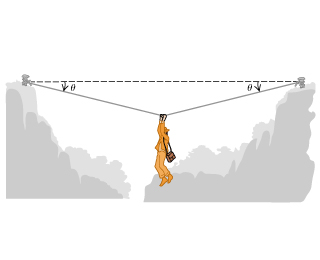# Problem: An adventurous archaeologist crosses between two rock cliffs by slowly going hand-over-hand along a rope stretched between the cliffs. He stops to rest at the middle of the rope. The rope will break if the tension in it exceeds 2.55×104 N, and our hero's mass is 87.6 kg .(a) If the angle between the rope and the horizontal is θ exttip{heta }{theta} = 11.1°, find the tension in the rope.(b) What is the smallest value the angle heta can have if the rope is not to break?

###### FREE Expert Solution
80% (486 ratings)
###### Problem Details

An adventurous archaeologist crosses between two rock cliffs by slowly going hand-over-hand along a rope stretched between the cliffs. He stops to rest at the middle of the rope. The rope will break if the tension in it exceeds 2.55×104 N, and our hero's mass is 87.6 kg .
(a) If the angle between the rope and the horizontal is θ = 11.1°, find the tension in the rope.
(b) What is the smallest value the angle can have if the rope is not to break?Frequently Asked Questions

What scientific concept do you need to know in order to solve this problem?

Our tutors have indicated that to solve this problem you will need to apply the Equilibrium in 2D concept. You can view video lessons to learn Equilibrium in 2D. Or if you need more Equilibrium in 2D practice, you can also practice Equilibrium in 2D practice problems.

How long does this problem take to solve?

Our expert Physics tutor, Julia took 4 minutes and 33 seconds to solve this problem. You can follow their steps in the video explanation above.

What professor is this problem relevant for?

Based on our data, we think this problem is relevant for Professor Justice's class at UC.